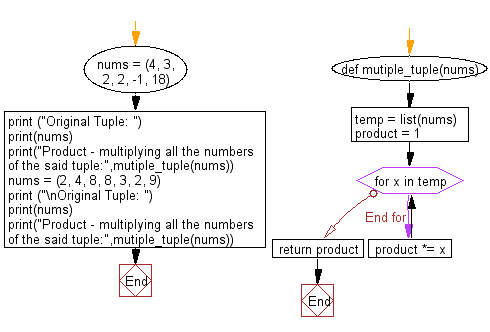﻿ Python: Calculate the product, multiplying all the numbers of a given tuple - w3resource# Python Exercise: Calculate the product, multiplying all the numbers of a given tuple

## Python tuple: Exercise-26 with Solution

Write a Python program calculate the product, multiplying all the numbers of a given tuple.

Sample Solution:-

Python Code:

``````def mutiple_tuple(nums):
temp = list(nums)
product = 1
for x in temp:
product *= x
return product

nums = (4, 3, 2, 2, -1, 18)
print ("Original Tuple: ")
print(nums)
print("Product - multiplying all the numbers of the said tuple:",mutiple_tuple(nums))

nums = (2, 4, 8, 8, 3, 2, 9)
print ("\nOriginal Tuple: ")
print(nums)
print("Product - multiplying all the numbers of the said tuple:",mutiple_tuple(nums))
```
```

Sample Output:

```Original Tuple:
(4, 3, 2, 2, -1, 18)
Product - multiplying all the numbers of the said tuple: -864

Original Tuple:
(2, 4, 8, 8, 3, 2, 9)
Product - multiplying all the numbers of the said tuple: 27648
```

Flowchart:## Visualize Python code execution:

The following tool visualize what the computer is doing step-by-step as it executes the said program:

Python Code Editor:

Have another way to solve this solution? Contribute your code (and comments) through Disqus.

What is the difficulty level of this exercise?

Test your Programming skills with w3resource's quiz.

﻿

## Python: Tips of the Day

Decapitalizes the first letter of a string:

Example:

```def tips_decapitalize(s, upper_rest=False):
return s[:1].lower() + (s[1:].upper() if upper_rest else s[1:])
print(tips_decapitalize('PythonTips'))
print(tips_decapitalize('PythonTips', True))
```

Output:

```pythonTips
pYTHONTIPS
```